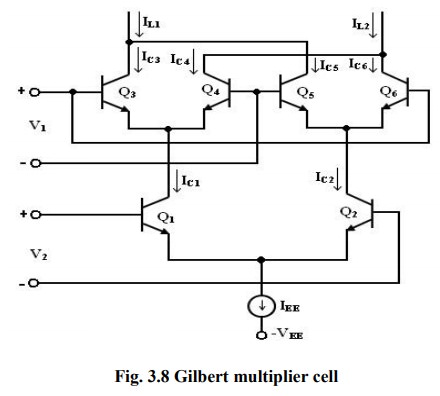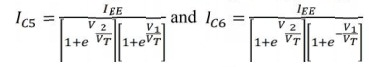Home | | Linear Integrated Circuits | Gilbert Multiplier cell

# Gilbert Multiplier cell

The Gilbert multiplier cell is a modification of the emitter coupled cell and this allows four – quadrant multiplication.

Gilbert Multiplier cell:

The Gilbert multiplier cell is a modification of the emitter coupled cell and this allows four – quadrant multiplication. Therefore, it forms the basis of most of the integrated circuit balanced Multipliers. Two cross- coupled emitter- coupled pairs in series connection with an emitter coupled pair form the structure of the Gilbert multiplier cell.The collector current of Q3 and Q4 are given bySimilarly, the collector current of Q5 and Q6 are given bycollector current IC1 and IC2 of transistors Q1 and Q2 can be expressed asSubstituting the above equation in IC3 and IC4, we getSimilarly substituting Ic2= in Ic5 and IC6 , we get,The differential output current I is given by

∆I =IL1 - IL2

= (IC3 +IC5) - ( IC4+IC6)

=IC3 - IC6)-(IC4 - IC5)

∆I=IEE tanh( V1/2VT) tanh(V2/2VT)

Tags : Analog Multiplier , Linear Integrated Circuits : Analog Multiplier and PLL
Study Material, Lecturing Notes, Assignment, Reference, Wiki description explanation, brief detail
Linear Integrated Circuits : Analog Multiplier and PLL : Gilbert Multiplier cell | Analog Multiplier